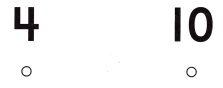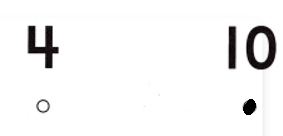# Texas Go Math Kindergarten Lesson 6.6 Answer Key Compare Numbers to 10

Refer to our Texas Go Math Kindergarten Answer Key Pdf to score good marks in the exams. Test yourself by practicing the problems from Texas Go Math Kindergarten Lesson 6.6 Answer Key Compare Numbers to 10.

## Texas Go Math Kindergarten Lesson 6.6 Answer Key Compare Numbers to 10

Unlock the Problem

DIRECTIONS: Carlos has 7 cubes. Jenny has one more cube than Carlos. How many cubes does Jenny have? Show the cubes. Compare the sets of cubes. Draw the cubes. Circle the set of cubes that has more.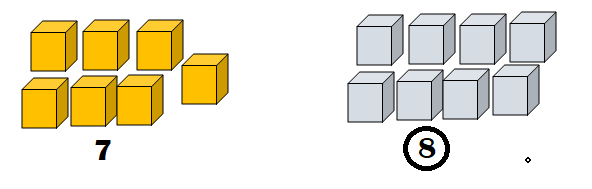Explanation:
Number of cubes Carlos has = 7.
Jenny has one more cube than Carlos.
=> Number of cubes Jenny has  = Number of cubes Carlos has + 1
= 7 + 1
= 8.

Try Another Problem

DIRECTIONS: 1. Paul has 3 blocks. Brody has two more blocks than Paul. Use cubes to model the sets of blocks. Compare the sets. Which set has more? Draw the cubes. Write how many in each set. Circle the greater number. Tell a friend how you compared the numbers.

Question 1.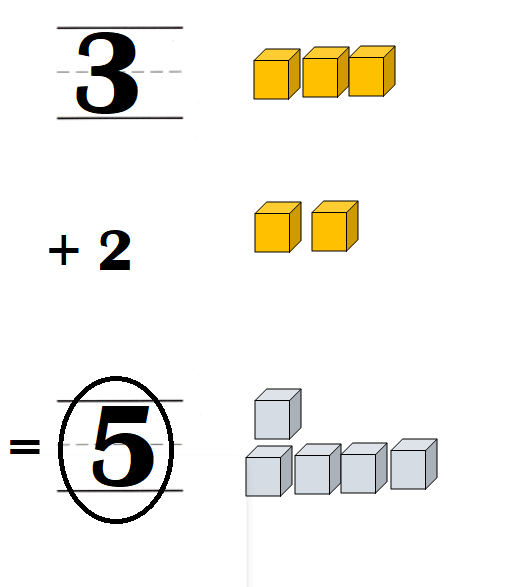Explanation:
Number of blocks Paul has = 3.
Brody has two more blocks than Paul.
Number of blocks Brody has = Number of blocks Paul has  + 2
= 3 + 2
= 5.

Share and Show

DIRECTIONS: 2. Libby has 7 beads. Madeline has three fewer beads than Libby. Use cubes to model the sets of beads. Compare the sets. Which set has fewer cubes? Draw the cubes. Write how many in each set. Circle the number that is less. Tell a friend how you compared the numbers.

Question 2.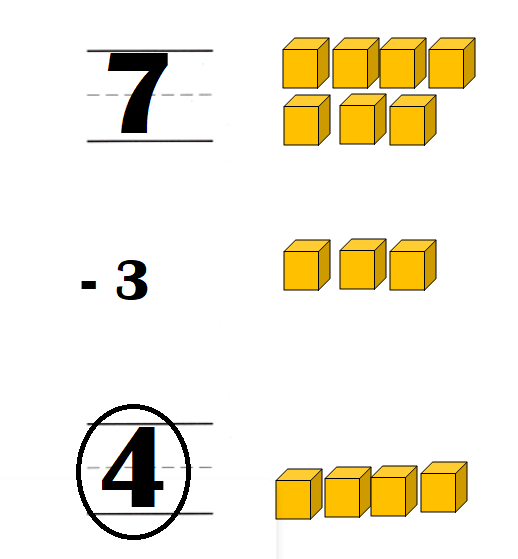Explanation:
Number of beads Libby has = 7.
= 7 – 3
= 4.

HOME ACTIVITY • Have your child count two sets of objects in your home, and write how many are in each set. Then have him or her circle the greater number. Repeat with sets of different numbers.Explanation:
My kid took plastic spoons and plastic glasses.
Number of plastic spoons = 8.
Number of plastic glasses = 5.
8 > 5.

DIRECTIONS: Choose the correct answer. 3. There are 6 ladybugs. Which is true about the number 6? 4. There are dogs. Which is true about the number 4? 5. Choose the correct answer. Which number is greater?

Question 3.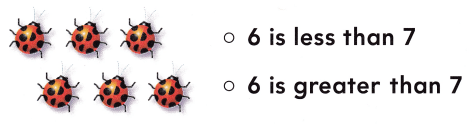Explanation:
Number of bugs = 6.
6 + 1 = 7.
=> 6 < 7 is true not 6 > 7.

Question 4.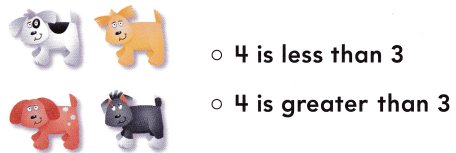Explanation:
Number of Puppies = 4.
4 – 1 = 3.
=> 4 > 3 is true not 4 < 3.

Question 5.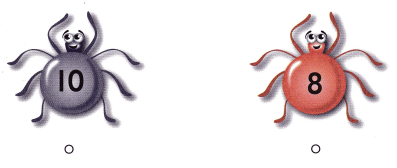Number 10 is greater than number 8.

Explanation:
Number 10 > Number 8.
=> 10 > 8 is true not 10 < 8.

### Texas Go Math Kindergarten Lesson 6.6 Homework and Practice Answer Key

DIRECTIONS: 1. Tony has 4 marbles. Joey has three more marbles than Tony. Draw to model the sets of marbles. Compare the sets. Which set has more marbles? Write how many in each set. Circle the greater number. Tell how you compared the numbers.

Question 1.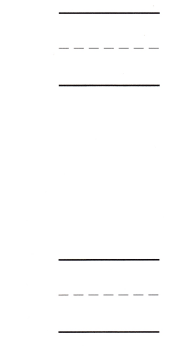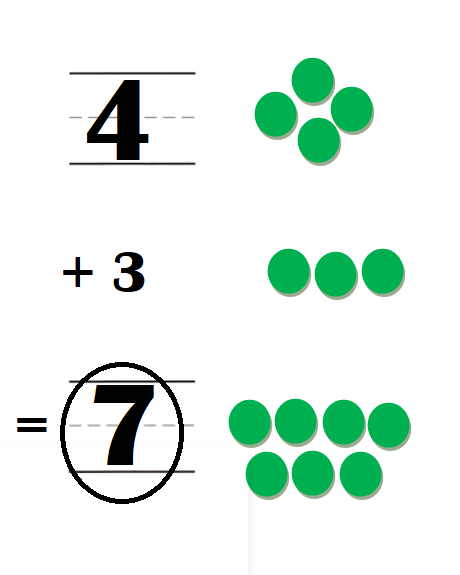Explanation:
Number of marbles Tony has = 4.
Joey has three more marbles than Tony.
Number of marbles Joey has = Number of marbles Tony has + 3
= 4 + 3
= 7.

DIRECTIONS: Choose the correct answer. 2. There are five bears. What is true about 5? 3. There are 7 bunnies. What is true about 7? 4. Which number is greater?

Lesson Check

Question 2.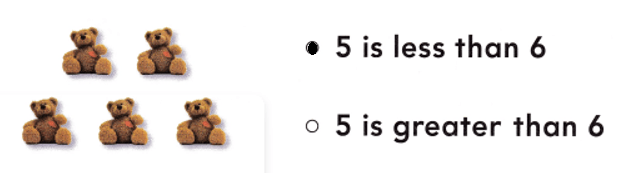Explanation:
Number of bears = 5.
5 + 1 = 6.
=> 5 < 6 is true not 5 > 6.

Question 3.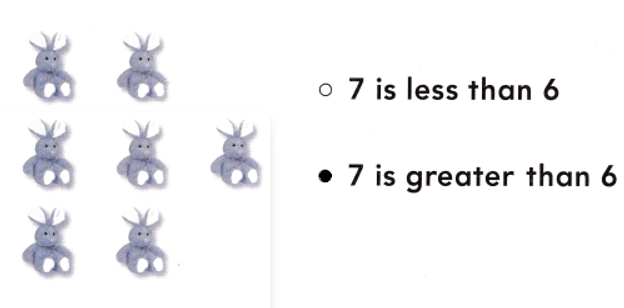Explanation:
Number of bunnies = 7.
7 – 1 = 6
=> 7 > 6 is true not 7 < 6.

Question 4.